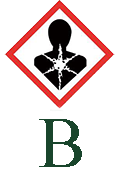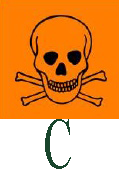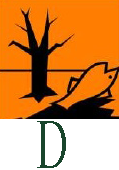# ল্যাবরেটরির নিরাপদ ব্যবহার-১উদ্দীপকটি লক্ষ কর এবং নিচের প্রশ্নটির উত্তর দাওতুমি ল্যাবরেটরিতে সংরক্ষণের ক্ষেত্রে ব্যবহার করবে-
$$i. \ H_{2}SO_{4}$$ এর পাত্রে $$C$$ চিহ্ন।
$$ii.$$ ল্যাবরেটরি বর্জ্যের পাত্রে $$D$$ চিহ্ন।
$$iii. \ Pb^{2+}, \ Hg^{2+}, \ Cd^{2+}$$ যৌগের পাত্রে $$B$$ চিহ্ন।
নিচের কোনটি সঠিক?

$$i$$
$$ii$$
$$i$$ ও $$ii$$
$$i,$$ $$ii$$ ও $$iii$$
উপরের কোনটি নয়

Calculator
Ans: $$i,$$ $$ii$$ ও $$iii$$## Multiple Choise

Physics Practice Basic
Chemistry Practice Basic
Zoology Practice Basic
Botany Practice Basic
English Practice Basic
General knowledge BCS Preparation
Mathematics BCS Preparation
Chemistry BCS Preparation
Botany BCS Preparation
Zoology BCS Preparation
Bangla BCS Preparation
English BCS Preparation
English BCS Preparation
Multiple Choise List
Mathematics Practice Basic
No post available in this Category !
Physics Practice Basic
No post available in this Category !
Chemistry Practice Basic
Zoology Practice Basic
No post available in this Category !
No post available in this Category !
Botany Practice Basic
No post available in this Category !
No post available in this Category !
English Practice Basic
No post available in this Category !
No post available in this Category !
General knowledge BCS Preparation
No post available in this Category !
No post available in this Category !
Mathematics BCS Preparation
No post available in this Category !
Physics BCS Preparation
Chemistry BCS Preparation
No post available in this Category !
No post available in this Category !
No post available in this Category !
No post available in this Category !
No post available in this Category !
No post available in this Category !